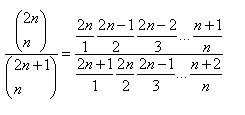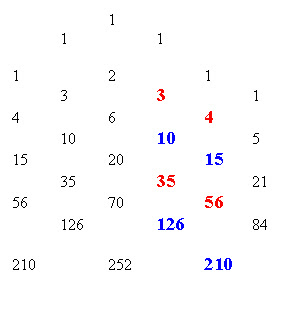## Sunday, 22 March 2009

### Probably an Interesting Problem

It was a simple probability problem, but it teased me with its surprising answer, and led me on … but let me start at the beginning…
A message on the AP discussion group from “Skerbie” (would I make this up?) asked…

If there are 4 red balls and 3 white balls in a container, and you remove them one at a time at random until one color is extinguished, what is the probability the remaining balls are red?

The simple answer, 4/7… soooo… would that always happen? And what is the math at work.

I quickly tried several examples where the number of white and red differed by one and they all seemed to preserve the ratio. At first I thought that it would only work with a difference of one, but with a wake up reminder from Joshua Zucker I corrected my arithmetic flaws and figured out that, Yes, the probability of the smaller color being extinguished first is the ratio of red/(red+white). SO… to show why….

One of the first things that I thought was interesting and (at least by me) unsuspected is that the probability of drawing WWW is the same as drawing WRWRW, or RRWWW, or RWWRW or any of the possible outcomes that ends with white being exhausted first. For the original seven problem case, the probability of drawing WWW is (3/7) (2/6)(1/5)= 1/35…The same probability applies to, for example, WRWRW which is (3/7)(4/6)(2/5)(3/4)(1/3) because the last two fractions simply cancel out the impact of the red and we get 1/35 again… I picked up pretty quickly that the 35 in the denominator was the combination of 7 things taken 3 at a time. I checked a few other starting quantities and this was true of the others as well, if you had n white balls, and n+d red balls, the probability of any of the paths ending with white being exhausted first led to a probability of 1 over 2n+d choose n.

As I looked at counting the possible outcomes ending in white being exhausted, I realized that :
There was only one way to draw WWW
There were three ways to draw them with one red in the mix if the red could not be at the end
RWWW; WRWW; WWRW
And there were six ways to draw them with two reds in the mix.
RRWWW, RWRWW; RWWRW; WRRWW; WRWRW; WWRRW
And there were ten ways to draw them with three reds in the mix… this was the sequence of triangular numbers, in particular I realized that if the last one HAD to be white, the sequence was counting 2 choose 2, and then 3 choose 1; 4 choose 2; 5 choose 3. But since all of those had the same probability of occurring, then the total probability was just the sum of those multiplied by 1/35. And the sum of the numbers down that diagonal of Pascal’s arithmetic triangle was 20, which is 6 choose 3….

I extended this to realize that as long as White had N balls, and Red had N+1, then there would be 2N choose N ways to order the outcomes, and each had a probability of 2N+1 choose N

In Pascal’s triangle, these were the successive numbers marked below:The first is for two reds and one white which leads to a probability of 2/3. The second is for three red and two white, and then for the four red, three white case. The 70/126 is the probability for starting with five red and four whites, and simplifies to the expected 5/9.

When we expand the values for 2N choose N and 2N+1 choose N,we see why the result always leads to the result that the overall probably of white being exhausted first is Red/(Red + White) which, when there are N+1 Reds and N whites leads to (N+1) / (2N+1) .

A very similar approach shows that when the difference between the number of Reds and Whites in the bowl is two, then we just move over one column in the arithmetic triangle to get :Ok, Maybe I’m the only old guy on the block who didn’t expect that…. But I bet a fistful of nickels that any bright kid in Alg II could look at what just happened and know where to go to find the answer if Red was three more than White…

They have the arithmetic triangle dating back to at least the thirteenth century, so why am I just finding this stuff out….